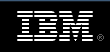All of IBM# Floating-Point Test Generator - FPgen

Unit Verification and Core TechnologiesTest Suite for IEEE 754R Compliance - Version 1.1

This document describes the contents of the first version of the IEEE 754R compliance test suite. For a full description of the structure, contents, and syntax of the test suite, please refer to the test suite document.

These test vectors are arranged in files. Each file represents the test vectors for a model described in the document.

Limitations of Version 1.02

This is a preliminary version, supporting only part of the features of the IEEE 754R standard.

Binary test suite:
• We generated tests only for operations whose inputs are in binary 32 format (single precision).
• In this version we only generated tests for tininess detection before rounding and for loss of accuracy detection as an inexact result.
• Operations included are addition, subtraction, multiplication, division, sqrt, convert float to float, copy, negate, abs, fused-multiply-add, is signed, is normal, is finite, is zero, is subnormal, is inf, is signaling, is nan, minnum, maxnum, minnummag, maxnummag.
• Please note that these files were created under UNIX, so some text editors might concatenate all the lines into one.

Decimal test suite:
• In this version we only generated tests for tininess detection before rounding and for loss of accuracy detection as an inexact result.
• Operations included are addition, subtraction, multiplication, division.
• Please note that these files were created under UNIX, so some text editors might concatenate all the lines into one.

Binary Models

Model Name Relevant Operations Actual Number of Tests
B1 Floating-Point basic types - inputs b32?f, b32?i, b32?N, b32?n, b32?sN, b32?-, b32?s, b32?0, b32A, b32cp, b32~, b32+, b32-, b32*, b32/, b32V, b32*+, b32>C, b32>A, b32<C,b32b128cff, b32b64cff 26166
Floating-Point basic types - intermediate b32+, b32-, b32*, b32/, b32V, b32*+ 214
B2 Near FP base values - hamming distance b32+, b32-, b32*, b32/, b32V, b32*+ 273
B3 Rounding - sign, LSB, guard bit, sticky bit b32+, b32-, b32*, b32/, b32V, b32*+ 652
B4 Overflow and near overflow b32+, b32-, b32*, b32/, b32V, b32*+ 2436
B5 Underflow and near underflow b32+, b32-, b32*, b32/, b32V, b32*+ 2672
B6 Corner rounding intermediate tasks b32*, b32/, b32*+ 256
B7 Sticky bit calculation b32+, b32-, b32*, b32*+ 98
B8 Vicinity of rounding boundaries b32+, b32-, b32*, b32/, b32*+ 656
B9 Special significands on inputs b32*, b32/, b32V 1190
B10 Add: shift b32+, b32- 114
B11 Add: shift combined with special significands b32+, b32- 32946
B12 Add: cancellation b32+, b32- 52
B13 Add: cancellation and subnorm result b32+, b32- 1192
B15 Multiply-add: shift combined with special significands b32*+ 21386
B17 Multiply-add: cancellation and subnorm result b32*+ 2252
B18 Multiply-add: special events - inexact b32*+ 11
Multiply-add: special events - overflow b32*+ 20
Multiply-add: special events - underflow b32*+ 40
B19 Compare: different input fields relations b32>C, b32>A, b32<C 317
B20 Divide: trailing zeros b32/, b32V 36
B21 Divide: divide by zero exception b32/ 32

Decimal Models

Model Name Relevant Operations Actual Number of Tests
D1 Decimal FP basic types - inputs d64+, d64-, d64*, d64/, d128+, d128-, d128*, d128/ 12544
Decimal FP basic types - intermediate d64+, d64-, d64*, d64/, d128+, d128-, d128*, d128/ 320
D3 Decimal FP - rounding d64+, d64-, d64*, d64/, d128+, d128-, d128*, d128/ 371
D4 Decimal FP - Overflow d64+, d64-, d64*, d64/, d128+, d128-, d128*, d128/ 2840
D5 Decimal FP - Underflow d64+, d64-, d64*, d64/, d128+, d128-, d128*, d128/ 2310
D11 Decimal FP - Trailing and leading zeros input d64+, d64-, d64*, d64/, d128+, d128-, d128*, d128/ 5648
D12 Decimal FP - Trailing and leading zeros result d64/, d128/ 2313
D15 Decimal FP - Clamping d64+, d64-, d64*, d64/, d128+, d128-, d128*, d128/ 4426
D24 Decimal FP - Mul Trailing Zeros d64*, d128* 6524